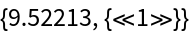#Function Repository Resource:

# EnumerateWolframModelRules

Enumerate canonical Wolfram model rules with a particular signature

Contributed by: Ed Pegg Jr and Max Piskunov
 ResourceFunction["EnumerateWolframModelRules"][signature] generates a list of all canonical connected Wolfram model rules with the specified signature. ResourceFunction["EnumerateWolframModelRules"][signature,s] allows only up to s distinct elements. ResourceFunction["EnumerateWolframModelRules"][signature,type] generates all possible rules with the specified type of connectivity. ResourceFunction["EnumerateWolframModelRules"][signature,{s,type}] uses up to s distinct elements, with specified connectivity type.

## Details and Options

Signatures are given in the form {{n1L,k1L},{n2L,k2L},}{{n1R,k1R},}.
The connectivity types can be:
 require both left- and right-hand sides to be connected individually and together require left-hand side to be connected, and connected to right-hand side do not constrain based on connectivity
The following option can be given:
 "Monitored" monitor computation
ResourceFunction["EnumerateWolframModelRules"] by default uses parallel computation.

## Examples

### Basic Examples

Show all Wolfram model rules with the signature 1212:

 In:=Out=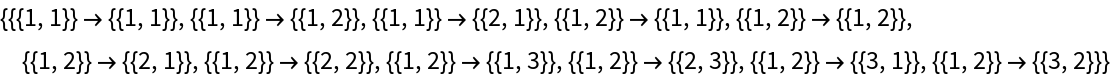### Scope

Wolfram model rules with signature 2212 and up to two distinct elements:

 In:=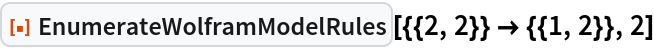Out=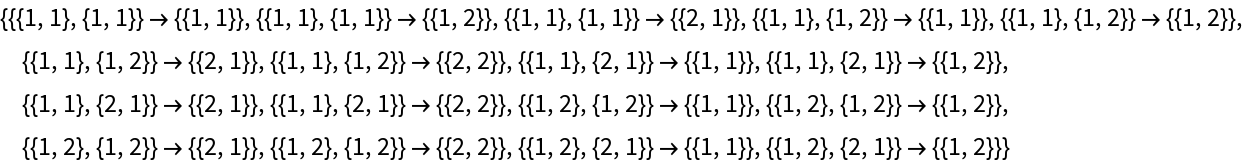Wolfram model rules with signature 2212 and no connectivity constraints:

 In:=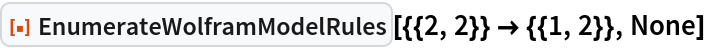Out=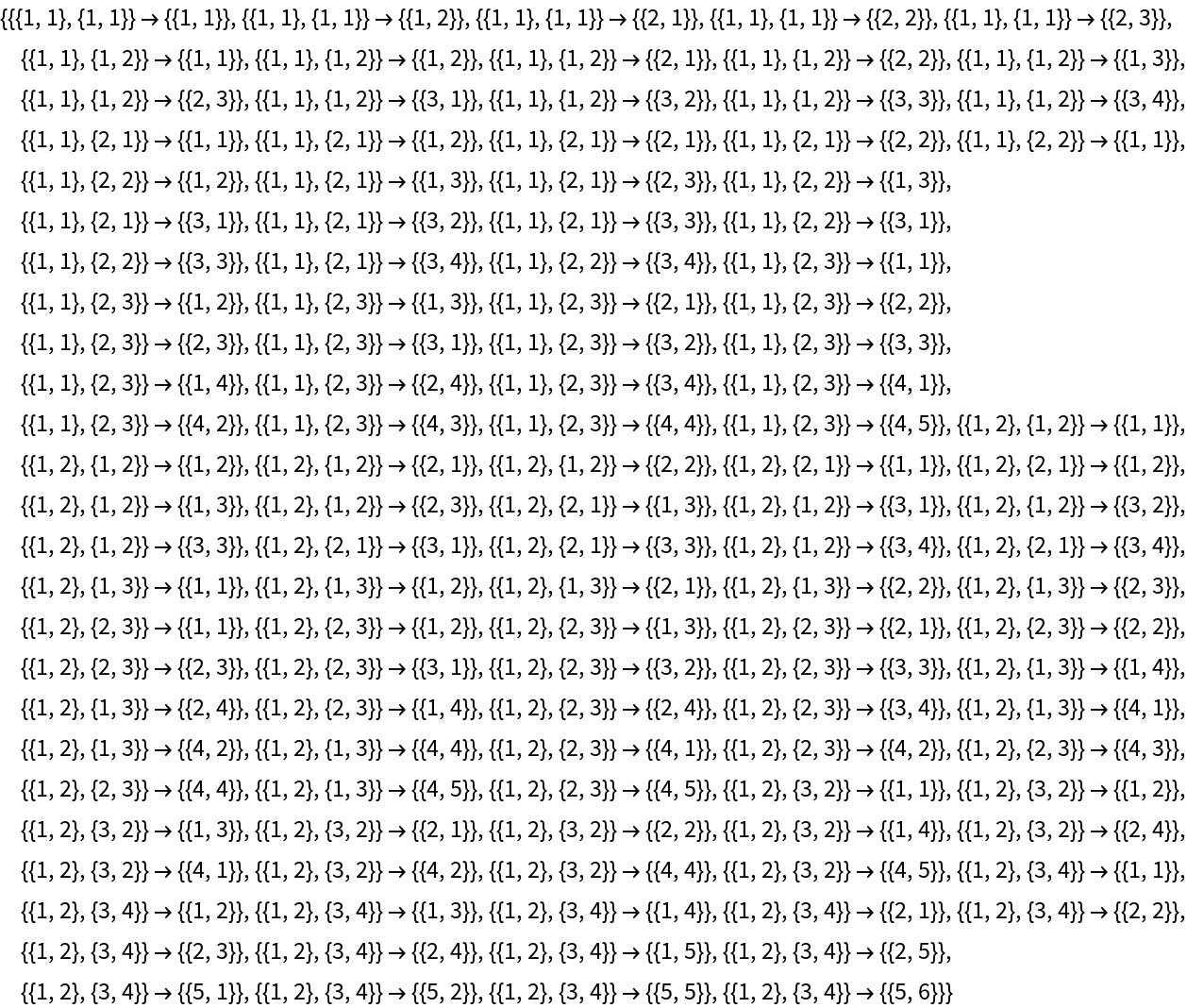Enumerate Wolfram model rules with signature 2212 and connected left- and right-hand sides:

 In:=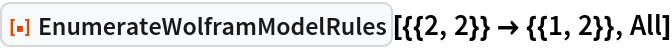Out=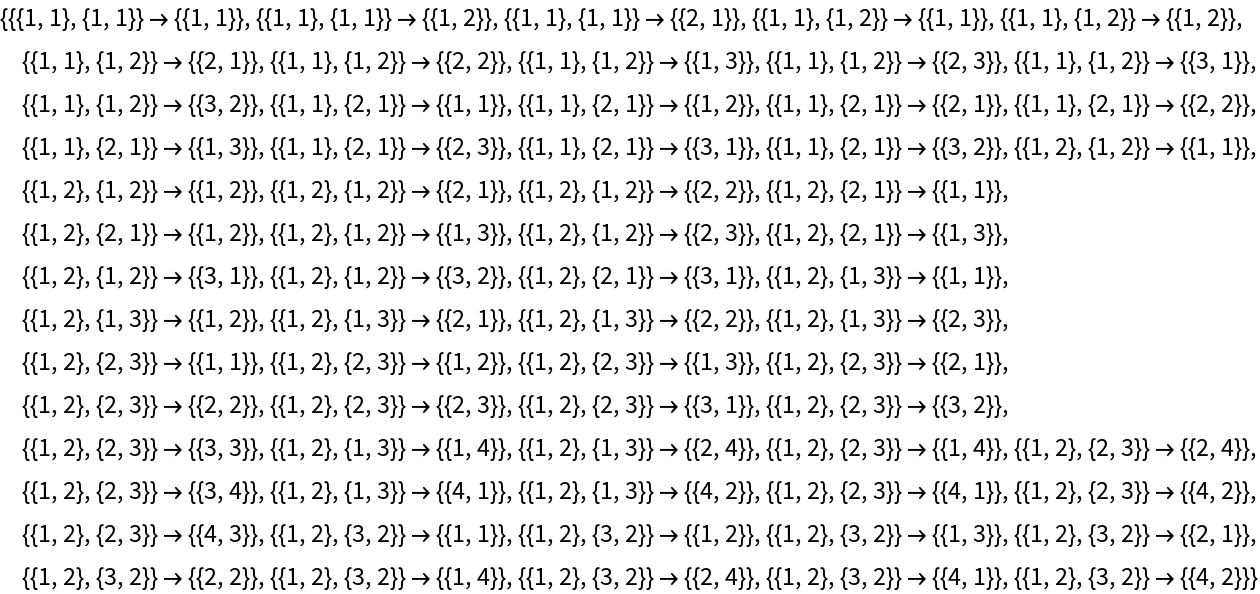### Possible Issues

Enumeration may take a long time even for moderately complicated signatures:

 In:=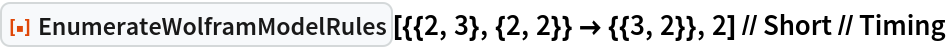Out=